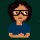Brainy Puzzles
Puzzle Solvers: Interesting brainy puzzles shared by fellow puzzlers
383 Members
Join this group to post and comment.Kaustubh Katdare • Dec 30, 2008

# IBM Puzzle December 2008 Challenge

Source: IBM Research | Ponder This | December 2008 challenges

Consider a random walk on the 2-d integer lattice starting at (0,0). From a lattice point (i,j) the walk moves to one of the four points (i-1, j), (i+1, j), (i, j-1) or (i, j+1) with equal probability. The walk continues until four different points (including (0,0)) have been visited. These four points will form one of the five tetrominoes (considering mirror images to be the same). For each tetromino find the probability that it will be the one formed in this way.

Please make sure you clearly identify the tetrominoes when submitting a solution. You may wish to use the wikipedia letter designations, I,L,O,S and T. A few solutions did not receive credit
because I could not tell which tetrominoes were which. Also I am requiring exact values for the probabilities.durga ch • Jan 3, 2009
I don't understand it much 😕Kaustubh Katdare • Jan 29, 2009
No one tried this one. Here is the solution -

Source: IBM Research | Ponder This | December 2008 solutions

XXX = 8/21
X

XX = 4/21
xx

XX = 4/21
xx

XXX = 3/21
x

XXXX = 2/21

They can be found by working out the transition probabilities from smaller forms. The calculations are simplified by taking symmetry into account. For example at each step the unique domino has a 1/2 probability of becoming a bent tromino, a 1/4 probability of becoming a straight tromino and a 1/4 probability of remaining a domino. So the random walk has a 2/3 probability of forming the bent tromino and a 1/3 probability of forming the straight tromino. Similarly one can compute the probability of transitioning from each tromino to each tetromino (keeping track of whether the walker is in the middle or at the end of the tromino). Then sum over the ways of forming each tetromino to find the values above. I omit the details.